# Copied!

May 25, 2020
After-Effects expression
Move in a square

Take the original position value and returns a point on a square around it. use "time" to drive the animation like in the example below.

Good Boy Ninja
All Tools Sale
For a limited time
9
Days
99
Hours
99
Minutes
99
Seconds
Promo ends 30 Aug, 20:00 UTC
Null Layer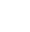Position
Expression

function SquareMotion(squareSize, percent) {

squareSize = (squareSize === undefined) ? 100 : squareSize;

percent = (percent === undefined) ? 0 : percent % 100;

sideSize = squareSize / Math.SQRT2;

halfSize = sideSize * 0.5;

perimeter = sideSize * 4; // convert the original percent value to both horizontal and vertical percent values to drive the motion

horizontalpercent = (percent >= 25 && percent <= 50) ? 100 : (percent >= 75 && percent <= 100) ? 0 : (percent < 25) ? (percent * 4) : (100 - (4 * (percent % 25)));

verticalpercent = (percent >= 0 && percent <= 25) ? 0 : (percent >= 50 && percent <= 75) ? 100 : (percent < 50) ? (percent - 25) * 4 : (100 - (4 * (percent % 25))); // use the linear function to drive each dimension

xLoc = linear(horizontalpercent, 0, 100, -halfSize, halfSize);

yLoc = linear(verticalpercent, 0, 100, -halfSize, halfSize);

return [xLoc, yLoc] + value;

}

squareSize = 400;

percent = time * 25;

SquareMotion(squareSize, percent)

Also check out...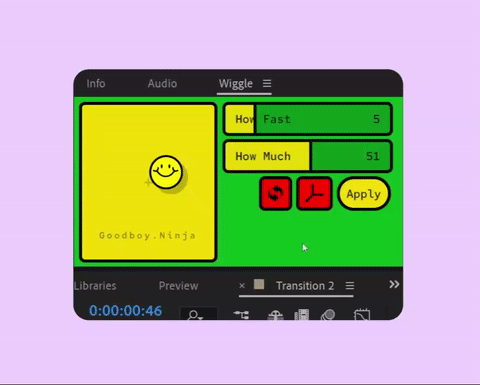Wiggle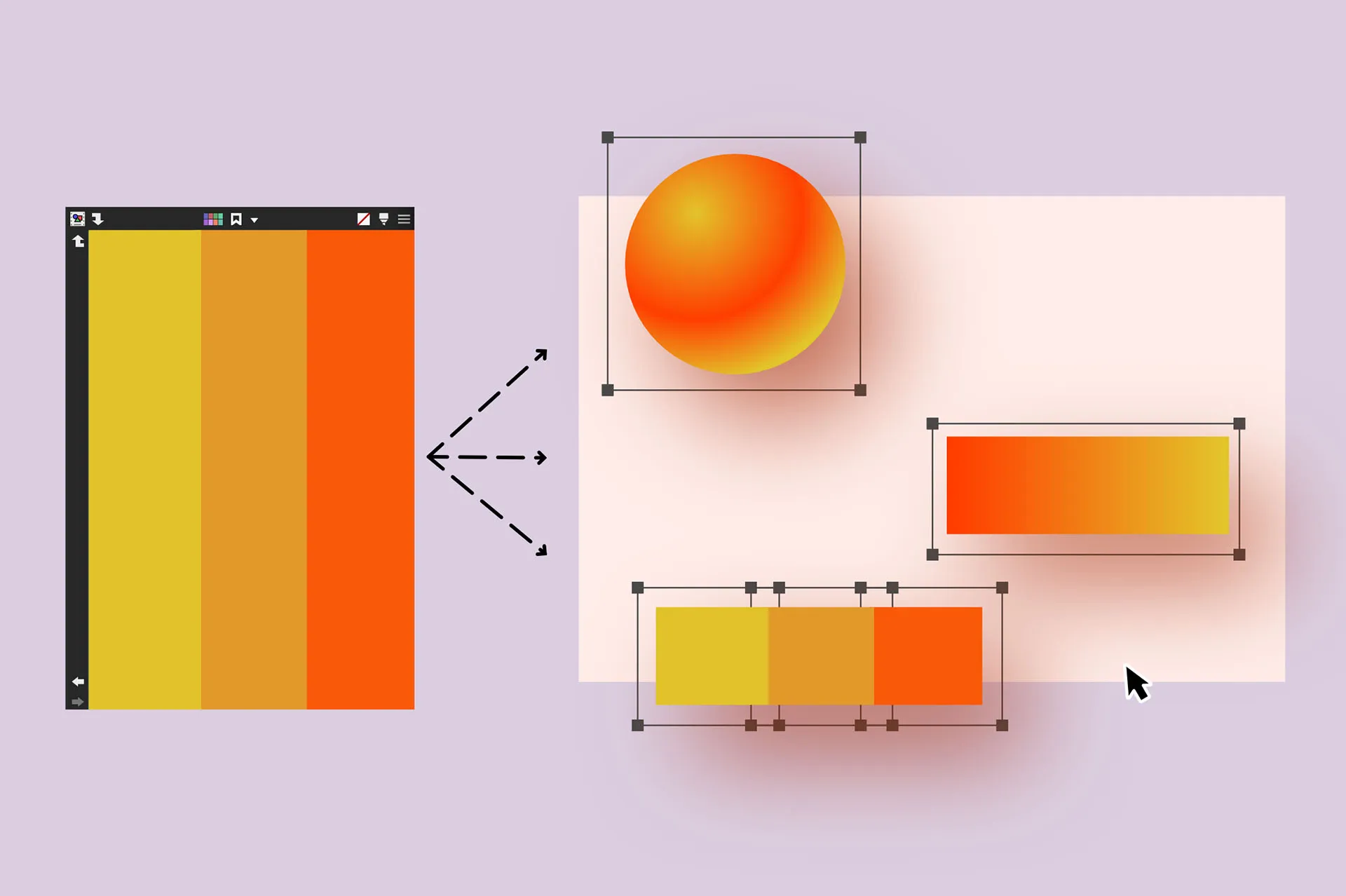ColorsNoodle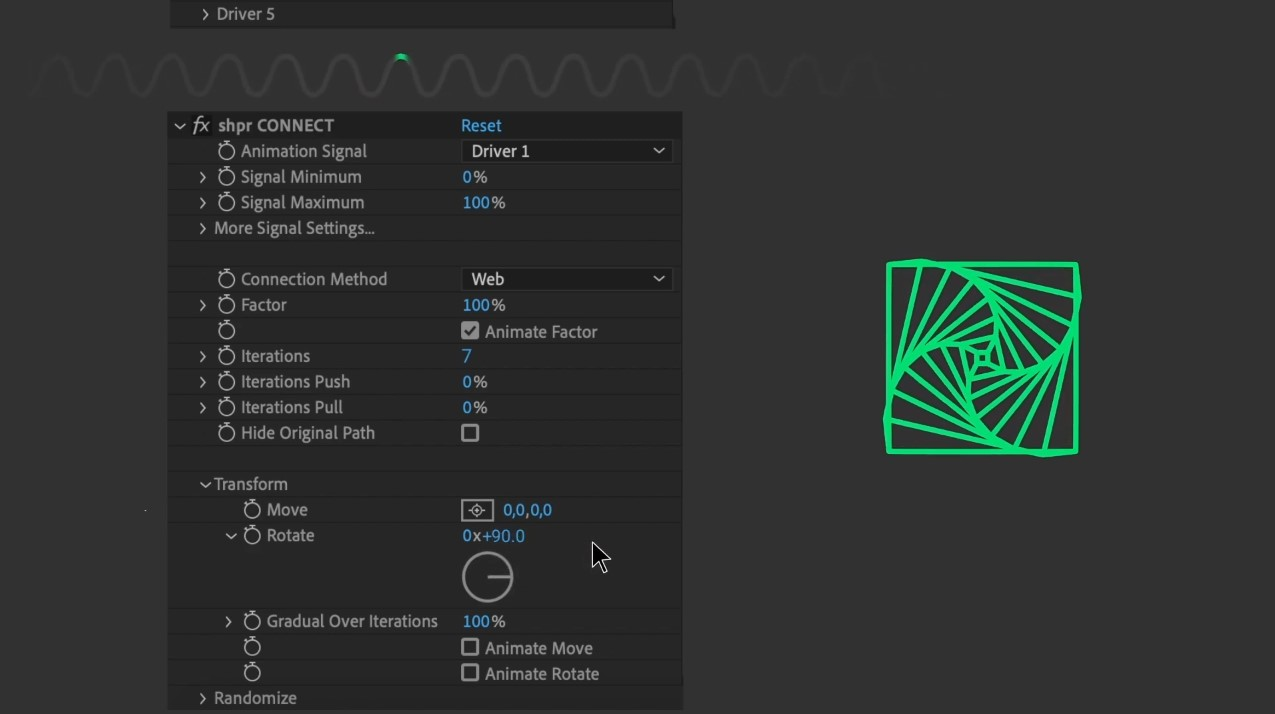shpr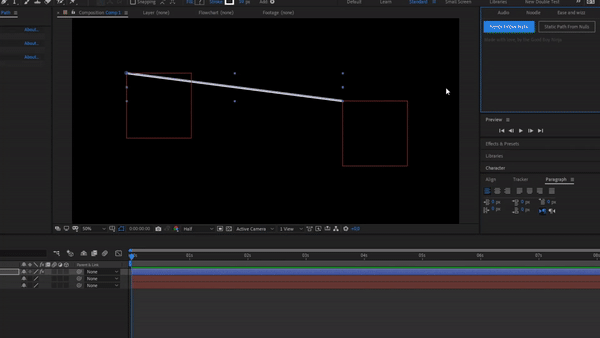Paths from Nulls